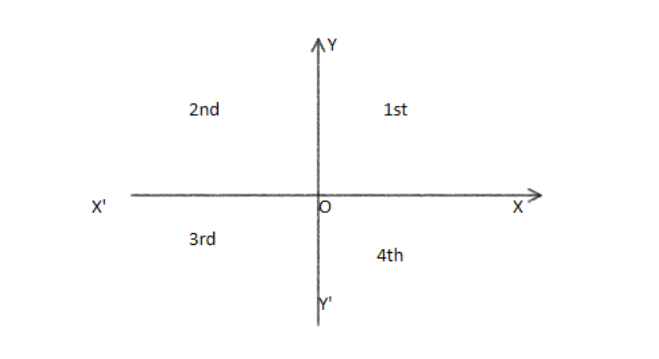Courses
Courses for Kids
Free study material
Offline Centres
MoreLast updated date: 01st Dec 2023
Total views: 277.8k
Views today: 6.77k

# Which of the following points lie in $III$ Quadrant?A. $\left( {1,3} \right)$ B. $\left( { - 1,3} \right)$ C. $\left( { + 1, - 3} \right)$ D. $\left( { - 1, - 3} \right)$Verified
277.8k+ views
Hint: To find which point lies in the 3rd quadrant, we are going to see the theory of the Cartesian plane. In the first quadrant, both x and y coordinates are positive. In the second quadrant, x coordinate is negative and y coordinate is positive. In the third quadrant, both the coordinates are negative and in the fourth quadrant x coordinate is positive and y coordinate is negative. So, the point from the given four points which has both x and y coordinates negative will be lying in the 3rd quadrant.

Complete step by step solution:
In this question, we are given 4 coordinates and we need to determine out of these 4 which coordinate lies in the 3rd quadrant. First of all, let us see a little theory about the Cartesian plane.
In mathematics, the Cartesian plane is defined as a 2dimensional coordinate plane formed by the intersection of the x-axis and y-axis.
This x-axis and y-axis split the plane into four parts called quadrants. Let us see the figure of a Cartesian plane.First quadrant is formed by the X and Y axis, so the values of x and y coordinates are positive in the 1st quadrant.
$\Rightarrow \left( {x,y} \right) = \left( { + , + } \right)$
Second quadrant is formed by the Y and X’ axis, so the value of y coordinate will be positive and the value of x coordinate will be negative in the 2nd quadrant.
$\Rightarrow \left( {x,y} \right) = \left( { - , + } \right)$
Third quadrant is formed by the X’ an Y’ axis, so the values of both x and y coordinates will be negative in the 3rd quadrant.
$\Rightarrow \left( {x,y} \right) = \left( { - , - } \right)$
$\Rightarrow \left( {x,y} \right) = \left( { + , - } \right)$
$\Rightarrow A\left( {1,3} \right) = \left( { + , + } \right)$
$\Rightarrow B\left( { - 1,3} \right) = \left( { - , + } \right)$
$\Rightarrow C\left( { + 1, - 3} \right) = \left( { + , - } \right)$
$\Rightarrow D\left( { - 1, - 3} \right) = \left( { - , - } \right)$
Therefore, we can see that point D has both the coordinates negative. Hence, $D\left( { - 3, - 1} \right)$ lies in the 3rd quadrant. Hence, option D is the correct answer.
Note: The ordered pair $\left( {a,b} \right)$ in the Cartesian plane is called the Cartesian coordinates. Here, the first number in the ordered pair is called abscissa. It is the distance of the point from the y axis. The second point in the ordered pair is called ordinate. It is the distance of the point from the x axis.